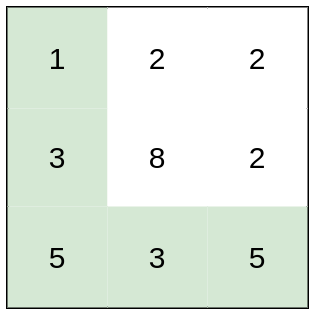New update is available. Click here to update.

# Path With Minimum Effort

Last Updated: 2 Apr, 2021
Difficulty: Hard

## PROBLEM STATEMENT

#### You are a Ninja training for an upcoming battle. You are given heights, a 2D array of size rows x columns, where heights[row][col] represents the height of a cell (which would contain a row and column). You are situated in the top-left cell, (0, 0), and you hope to travel to the bottom-right cell, (rows-1, columns-1) (i.e., 0-indexed). You can move up, down, left, or right, and you wish to find a route that requires the minimum time. A route's time is the maximum absolute difference in heights between two consecutive cells of the route. Return the minimum time required to travel from the top-left cell to the bottom-right cell.

##### For Example``````Input: heights = [[1,2,2],[3,8,2],[5,3,5]]
Output: 2
Explanation: The route of [1,3,5,3,5] has a maximum absolute difference of 2 in consecutive cells.
This is better than the route of [1,2,2,2,5], where the maximum absolute difference is 3.
``````
##### Input Format:
``````The first line determines the number of test cases that would be given in the problem.

The first line of each test case contains two space-separated integers where the first value is the number of rows ‘rows’ while the second integer is the number of columns ‘columns’ given for the 2-D list (array) heights

Now the 2-D list (array) input is taken according to the rows and columns entered before. I.e. The number of lines of input would be equal to the number of rows while in each line there would be as many space-separated integers as the number of columns for each row.

For example for a given row = 3 and columns = 3 we would have 3 lines of input with each line comprising of 3 space-separated integers.
``````
##### Output Format:
``````For every test case, Return the minimum time required by you to travel from the top-left cell to the bottom-right cell.

For each test case, print the output in a separate line.
``````
##### Constraint :
``````rows == heights.length
columns == heights[i].length
1 <= rows, columns <= 100
1 <= heights[i][j] <= 10^6

Where ‘rows’ is the number of rows and ‘columns’ is the number of columns. The 2-D list (array) heights would contain the cells and their values with a dimension of ‘rows’ x ‘columns’.

Time Limit: 1 sec
``````## Approach 1

The main idea here is to implement a dfs function with parameter TIME_LIMIT here: it is the maximum time you (as a ninja) can deal with. Then the idea is to use binary search to find the smallest TIME_LIMIT for which you can reach the ending point.

The algorithm will be-

1. Initialise the lengths of rows, columns and a neighbours 2-D list (array) comprising the valid set of moves which can be performed on the heights list (array).
2. Now we define a function named DFS(TIME_LIMIT, x, y), where TIME_LIMIT is the maximum time we can take to reach our destination and x, y are current coordinates for each iteration. To perform the depth-first search traversal, we need to visit all neighbours, and make sure that we follow the below constraints:
1. Do not go outside our grid
2. The cell is not visited yet
3. The effort to go from the old cell to a new one is less or equal to TIME_LIMIT.
3. Now we use a binary search to ensure that:
1. We can be sure that if TIME_LIMIT = -1, we never reach the end, and if TIME_LIMIT is maximum overall heights, then we will reach the end.
2. Choose the middle point and keep marking visited sets of cells, perform a depth first search and choose a left or right part to search for.
4. We finally return the minimum time taken to traverse from source to destination.